NEET  >  Test: Nuclear Chemistry - From Past 31 Years Questions

# Test: Nuclear Chemistry - From Past 31 Years Questions

Test Description

## 20 Questions MCQ Test Chemistry 31 Years NEET Chapterwise Solved Papers | Test: Nuclear Chemistry - From Past 31 Years Questions

Test: Nuclear Chemistry - From Past 31 Years Questions for NEET 2023 is part of Chemistry 31 Years NEET Chapterwise Solved Papers preparation. The Test: Nuclear Chemistry - From Past 31 Years Questions questions and answers have been prepared according to the NEET exam syllabus.The Test: Nuclear Chemistry - From Past 31 Years Questions MCQs are made for NEET 2023 Exam. Find important definitions, questions, notes, meanings, examples, exercises, MCQs and online tests for Test: Nuclear Chemistry - From Past 31 Years Questions below.
Solutions of Test: Nuclear Chemistry - From Past 31 Years Questions questions in English are available as part of our Chemistry 31 Years NEET Chapterwise Solved Papers for NEET & Test: Nuclear Chemistry - From Past 31 Years Questions solutions in Hindi for Chemistry 31 Years NEET Chapterwise Solved Papers course. Download more important topics, notes, lectures and mock test series for NEET Exam by signing up for free. Attempt Test: Nuclear Chemistry - From Past 31 Years Questions | 20 questions in 40 minutes | Mock test for NEET preparation | Free important questions MCQ to study Chemistry 31 Years NEET Chapterwise Solved Papers for NEET Exam | Download free PDF with solutions
 1 Crore+ students have signed up on EduRev. Have you?
Test: Nuclear Chemistry - From Past 31 Years Questions - Question 1

### The half life of a radioactive nuclide is 100 hours. The fraction of original activity that will remain after 150 hours would be:    

Detailed Solution for Test: Nuclear Chemistry - From Past 31 Years Questions - Question 1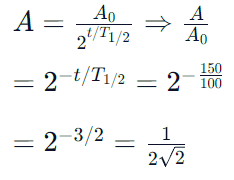Test: Nuclear Chemistry - From Past 31 Years Questions - Question 2

### The half-life of a radioactive sample undergoing α-decay is 1.4 × 1017 sec. If the number of nuclei in the sample is 2.0 × 1021, the activity of the sample is nearly equal to:  

Detailed Solution for Test: Nuclear Chemistry - From Past 31 Years Questions - Question 2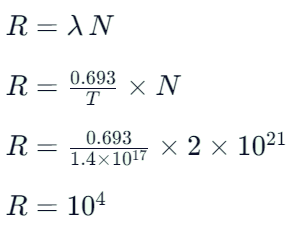Test: Nuclear Chemistry - From Past 31 Years Questions - Question 3

### The half life of a substance in a certain enzymecatalysedreaction is 138s. The time required forthe concentration of the substance to fall from1.28 mg L–1 to 0.04 mg L–1, is : 

Detailed Solution for Test: Nuclear Chemistry - From Past 31 Years Questions - Question 3

For a first order reaction
Total time T = no. of half lives (n) × half life
(t1/2)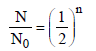where n = no. of half lives
Give N0 (original amount) = 1.28 mg/ ℓ
N (amount of substance left after time T)
= 0.04 m/g l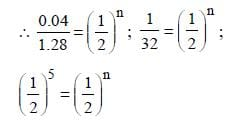n = 5
T = 5 × 138
= 690

Test: Nuclear Chemistry - From Past 31 Years Questions - Question 4

A nuclide of an alkaline earth metal undergoesradioactive decay by emission of the α-particles in succession. The group of theperiodic table to which the resulting daughterelement would belong is 

Detailed Solution for Test: Nuclear Chemistry - From Past 31 Years Questions - Question 4

When IIA group element (Ra) emits one
α-particle its group no. decreases by two
unit. i.e., go into zero group (Gr. 16) But as
it is radioactive thus due to successive
emission last product is Pb i.e., (Gr.14).

Test: Nuclear Chemistry - From Past 31 Years Questions - Question 5

The radioactive isotope, tritium,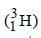has a halflifeof 12.3 years. If the initial amount of tritium is32 mg, how many milligrams of it would remainafter 49.2 years? 

Detailed Solution for Test: Nuclear Chemistry - From Past 31 Years Questions - Question 5

Given t1/2 = 12.3 years
Initial amount (N0) = 32 mg
Total time = 49.2 years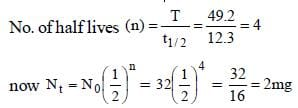Hence 32 mg becomes 2 mg in 49.2 years

Test: Nuclear Chemistry - From Past 31 Years Questions - Question 6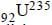nucleus absorbs a neutron and disintegrates into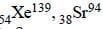and x. So what will be the product x? 

Detailed Solution for Test: Nuclear Chemistry - From Past 31 Years Questions - Question 6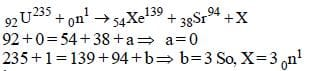i.e 3 neutrons

Test: Nuclear Chemistry - From Past 31 Years Questions - Question 7

A human body required 0.01M activity ofradioactive substance after 24 hours. Half life ofradioactive substance is 6 hours. Then injectionof maximum activity of radioactive substance thatcan be injected will be 

Detailed Solution for Test: Nuclear Chemistry - From Past 31 Years Questions - Question 7

Remaining activity = 0.01M
after 24 hrs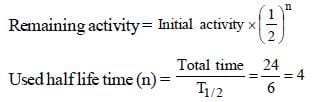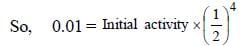Initial activity = 0.01 × 16 = 0.16M

Test: Nuclear Chemistry - From Past 31 Years Questions - Question 8

When a radioactive element emits successivelyone α-particle and two β-particles, the massnumber of the daughter element 

Detailed Solution for Test: Nuclear Chemistry - From Past 31 Years Questions - Question 8

Mass number is effected by emmision of α
particle while β particle has negligible mass
does not effect mass number. e.g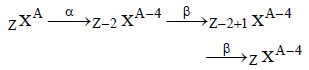Test: Nuclear Chemistry - From Past 31 Years Questions - Question 9

Carbon - 14 dating method is based on the factthat: 

Detailed Solution for Test: Nuclear Chemistry - From Past 31 Years Questions - Question 9

By carbon dating method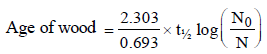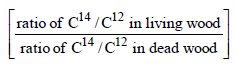Hence it is based upon the ratio of C14 and
C12.

Test: Nuclear Chemistry - From Past 31 Years Questions - Question 10

One microgram of radioactive sodium  2411Na witha half-life of 15 hours was injected into a livingsystem for a bio-assay. How long will it take forthe radioactive subtance to fall up to 25% of theinitial value? 

Detailed Solution for Test: Nuclear Chemistry - From Past 31 Years Questions - Question 10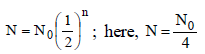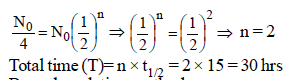Test: Nuclear Chemistry - From Past 31 Years Questions - Question 11

Half-life for radioactive 14C is 5760 years. In howmany years, 200 mg of 14C will be reduced to 25mg?           

Detailed Solution for Test: Nuclear Chemistry - From Past 31 Years Questions - Question 11

Half-life of 14C = 5760 yrs; Initial weight of
14C = 200 mg and final weight of 14C = 25 mg.
Quantity left after 5760 years = 200/2  = 100 mg

Similarly quantity left after another 5760
years (i.e 11520 years) = 100/2 = 50 mg

Quantity left after another 5760 years
(i.e. 17280 years) = 50/2 = 25 mg

Thus time taken by 200 mg of 14C to reduce
to 25 mg = (5760 + 5760 + 5760 ) years = 17280
years.

Alternative solution

As we know that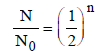where N0 original amount of radioactive
sustacnce
N = Amount of substance remain after n half
lives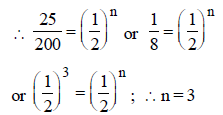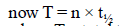where T = total time
T = 3 × 5760 years = 17280 years

Test: Nuclear Chemistry - From Past 31 Years Questions - Question 12

India has the world’s largest deposits of thoriumin the form of          

Detailed Solution for Test: Nuclear Chemistry - From Past 31 Years Questions - Question 12

The ore of thorium is monazite.

Test: Nuclear Chemistry - From Past 31 Years Questions - Question 13

In a radioactive decay, an emitted electron comesfrom 

Detailed Solution for Test: Nuclear Chemistry - From Past 31 Years Questions - Question 13

When a radioactive elements emits α or β
particle the new element formed may have
unstable nucleus. It may further disintegrate
by emitting α- or β particle forming a new
element. This process of integration may
continue till end product formed is a stable
compound.

Test: Nuclear Chemistry - From Past 31 Years Questions - Question 14

If an isotope of hydrogen has two neutrons in itsatom, its atomic number and atomic mass numberwill respectively be    

Detailed Solution for Test: Nuclear Chemistry - From Past 31 Years Questions - Question 14

As number of neutron = Mass number
– atomic number
Give number of neutron = 2
∴ Mass number will be 3 and atomic number
will be one.

Test: Nuclear Chemistry - From Past 31 Years Questions - Question 15

Emission of an alpha particle leads to a



Detailed Solution for Test: Nuclear Chemistry - From Past 31 Years Questions - Question 15

Emission of α -particle )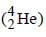leads to
decrease of 2 units of charge. e.g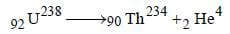Test: Nuclear Chemistry - From Past 31 Years Questions - Question 16

The age of most ancient geological formations is estimated by

Test: Nuclear Chemistry - From Past 31 Years Questions - Question 17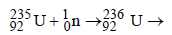fission products + neutrons + 3.20 × 10–11 J The energy released when 1 g of     finally undergoes fission is

Detailed Solution for Test: Nuclear Chemistry - From Past 31 Years Questions - Question 17

1 atom of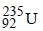on fission gives energy
= 3.2 × 10–11 J
6.023 × 1023 atom (1 mole) on fission gives
energy = 3.2 × 10–11 × 6.023 × 1023 J
235 gm ofon fission gives energy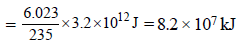Test: Nuclear Chemistry - From Past 31 Years Questions - Question 18

Number of neutrons in a parent nucleus X, which  gives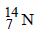nucleus, after two successive β emissions, would be

Detailed Solution for Test: Nuclear Chemistry - From Past 31 Years Questions - Question 18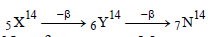No. of neutrons = Mass number – No. of
proton =14 – 5 = 9

Test: Nuclear Chemistry - From Past 31 Years Questions - Question 19

If species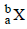emits firstly a positron, then two α and two β and in last one α and finally converted to species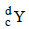, so correct relation is

Detailed Solution for Test: Nuclear Chemistry - From Past 31 Years Questions - Question 19Test: Nuclear Chemistry - From Past 31 Years Questions - Question 20

The radioactive isotope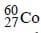which is used in the treatment of cancer can be made by (n, p) reaction. For this reaction the target nucleus is

Detailed Solution for Test: Nuclear Chemistry - From Past 31 Years Questions - Question 20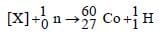Balancing the mass and atomic numbers on
both sidesThus X should be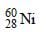## Chemistry 31 Years NEET Chapterwise Solved Papers

29 docs|52 tests
 Use Code STAYHOME200 and get INR 200 additional OFF Use Coupon Code
Information about Test: Nuclear Chemistry - From Past 31 Years Questions Page
In this test you can find the Exam questions for Test: Nuclear Chemistry - From Past 31 Years Questions solved & explained in the simplest way possible. Besides giving Questions and answers for Test: Nuclear Chemistry - From Past 31 Years Questions, EduRev gives you an ample number of Online tests for practice

## Chemistry 31 Years NEET Chapterwise Solved Papers

29 docs|52 tests

### How to Prepare for NEET

Read our guide to prepare for NEET which is created by Toppers & the best Teachers